Chapter 2 - Relations and Functions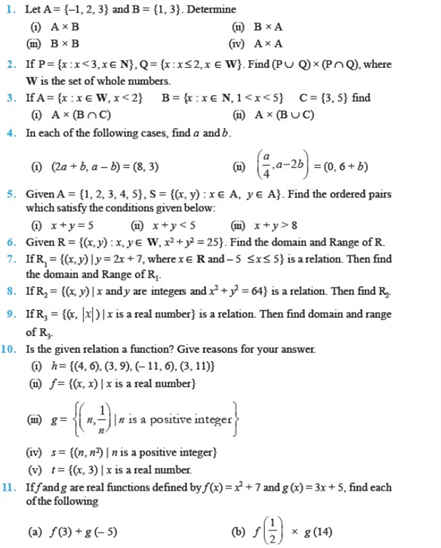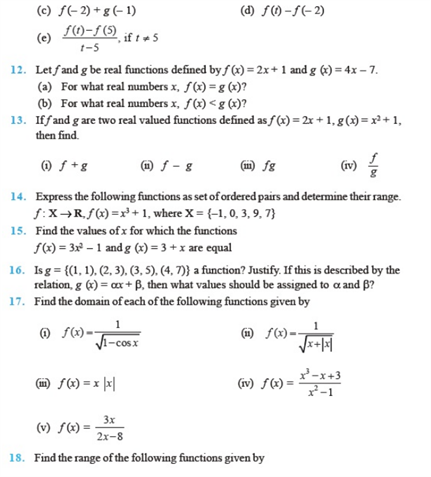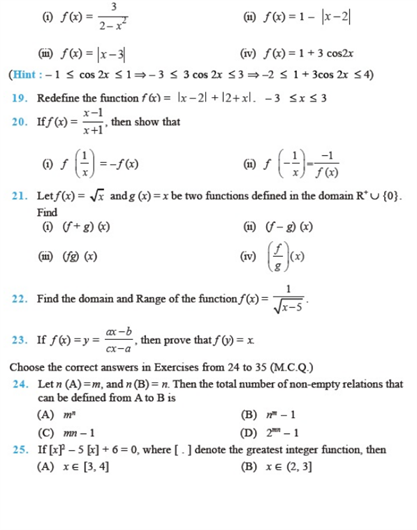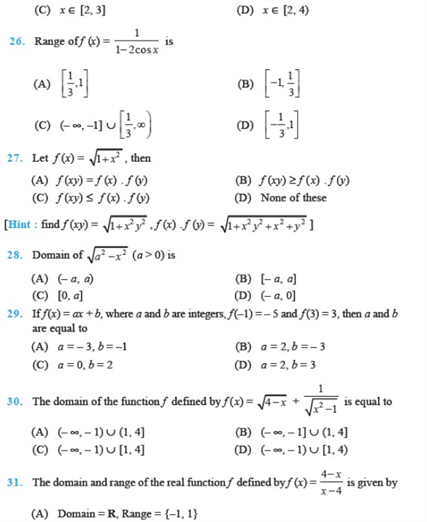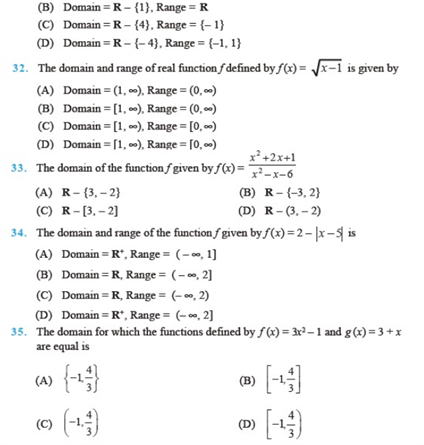Practise This Question

Let F1 be the set of all parallelograms, F2 the set of rectangles, F3 the set of rhombuses, F4 the set of squares and F5 the set of trapeziums in a plane, then F1 is equal to